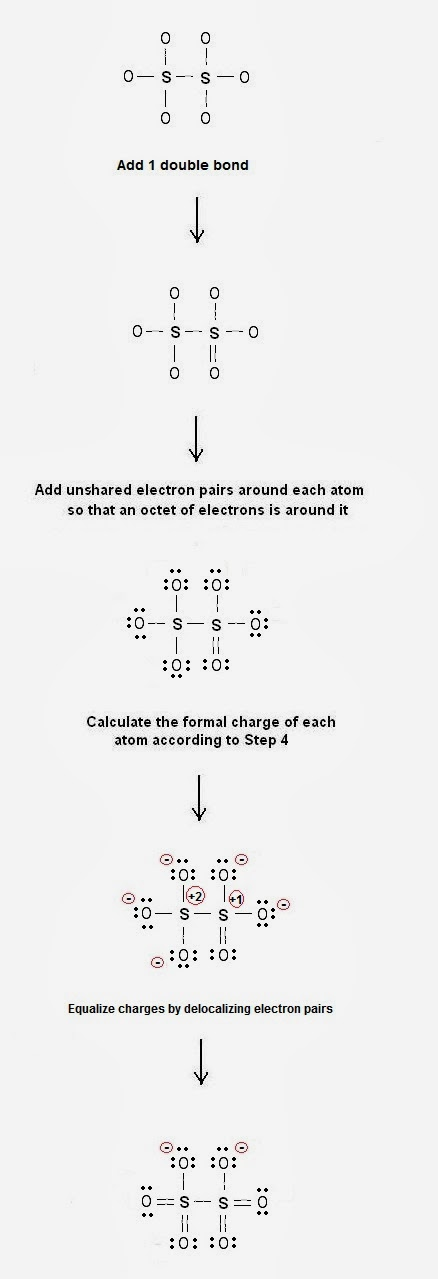Lewis Electron - Dot Structure of Dithionate S2O6 | Chemistry Net

# Lewis Electron - Dot Structure of Dithionate S2O6

A simple procedure for writing Lewis dot structures was given in a previous post entitled “Lewis Structures and the Octet Rule”.
Several worked examples relevant to this procedure were given in previous posts please see the Sitemap - Table of Contents (Lewis Electron Dot Structures).

Let us consider the case of Dithionate S2O6

Step 1: The central atoms is the S atoms. Connect the atoms with single bonds.Fig. 1: Connect the dithionate atoms with single bonds

Step 2: Calculate the # of electrons in π bonds (multiple bonds)  using  formula (1)

Where n in this case is 8. Where V = 6*6 + 2*6 = 48 , V is the number of valence electrons of the compound.
Therefore, P = 6n + 2 – V = 6 * 8 + 2 – 48  = 2   \  So, there is 1 double bond

Step 3 & 4: The  Lewis dot resonance structures of  S2O6  are as follows:Fig. 2: Plausible Lewis electron dot structures of dithionate